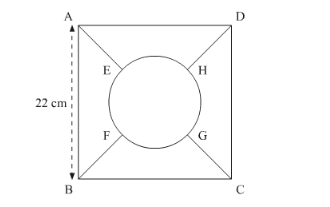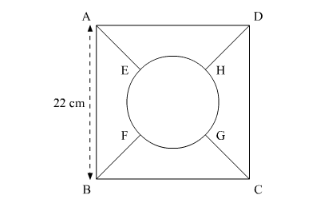# In the following figure, the square ABCD is divided into five equal parts,Question:

In the following figure, the square ABCD is divided into five equal parts, all having same area. The central part is circular and the lines AEGCBF and HD lie along the diagonals AC and BD of the square. If AB = 22 cm, find:

(i) the circumference of the central part.

(ii) the perimeter of the part ABEF.Solution:

We have a square ABCD.We have,

$\mathrm{AB}=22 \mathrm{~cm}$

(i)We have to find the perimeter of the triangle. We have a relation as,

Area of circular region $=\frac{1}{5}($ Area of $\mathrm{ABCD})$

So,

$\pi r^{2}=\frac{1}{5}(22)^{2}$

$r=\frac{22}{\sqrt{5 \pi}}$

$=5.56$

So perimeter of the circular region,

$=2 \pi r$

$=(2) \frac{22}{7}\left(\frac{22}{\sqrt{5 \pi}}\right)$

$=34.88 \mathrm{~cm}$

(ii)We have to find the perimeter of ABEF. Let O be the centre of the circular region.

Use Pythagoras theorem to get,

$2(\mathrm{AE}+r)^{2}=22^{2}$

$\mathrm{AE}+r=15.56$

$\mathrm{AE}=(15.56-5.56) \mathrm{cm}$

$=10 \mathrm{c}$,

Similarly,

$\mathrm{BF}=10 \mathrm{~cm}$

Now length of arc EF,

$=\frac{\text { Perimeter of circular region }}{4}$

$=\frac{34.88}{4} \mathrm{~cm}$

$=8.64 \mathrm{~cm}$

So, perimeter of ABFE,

$=\mathrm{AB}+\mathrm{BF}+\mathrm{EF}+\mathrm{AE}$

$=(22+10+8.64+10) \mathrm{cm}$

$=50.64 \mathrm{~cm}$